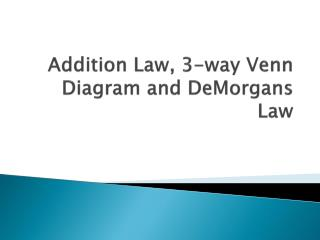# Addition Law, 3-way Venn Diagram and DeMorgans Law - PowerPoint PPT PresentationDownload PresentationAddition Law, 3-way Venn Diagram and DeMorgans Law

Addition Law, 3-way Venn Diagram and DeMorgans LawDownload Presentation## Addition Law, 3-way Venn Diagram and DeMorgans Law

- - - - - - - - - - - - - - - - - - - - - - - - - - - E N D - - - - - - - - - - - - - - - - - - - - - - - - - - -
##### Presentation Transcript

1. Addition Law, 3-way Venn Diagram and DeMorgans Law

2. We have talked about… • 1. Some concepts of probabilities: set, experiment, sample space, event, … • 2. Complement: A and Ac • and: P(A) + P(Ac)=1. • 3. Venn Diagram

3. How to find the probability of an event • In the easiest case, we first count the number of outcomes in the sample space, assuming each outcome is equally likely, then count the number of outcomes for the event of interest, do the division. • Example: • Event A={Roll a fair die and get at least 4} • Sample space {1,2,3,4,5,6} , 6 outcomes • Outcomes for this event: {4,5,6}, 3 outcomes • P(A)=3/6=0.5.

4. Addition Law • From Venn diagram, we deal with the probability associated with two or more events. • Using two events, A and B as an example. • We may be interested in A happens, B happens, A or B happens, A and B happens, A happens but B does not, B happens but A does not.

5. Addition Law • A or B happens, P(A U B), it is called union of A and B • Both A and B happens, P(A∩B), it is called the intersection of A and B. • Addition law: P(A U B) = P(A) + P(B) - P(A∩B).

6. Midterm Example, revisited • A class of 30 students took 2 midterms during a semester. 22 of them passed the first one and 26 of them passed the second one. If 3 students failed both, find the number of students who passed the each of the two midterms and failed the other. • Answer: 21 passed both, 1 passed the first but failed the second, 5 passed the second but failed the first, 3 failed both.

7. Addition Law • Think about the midterm example: • A={Pass midterm 1}; B={Pass midterm 2} • P(A∩B) = ? • P(A∩B) ={ Pass both exam 1 and 2} • P(A U B)=? • P(A U B) ={ Pass at least ONE exam }

8. Addition Law • Think about • P(A+B) or P(AUB)= P(A)+P(B)- P(A∩B) • Or, probability that one passes at least one exam = probability that one passes midterm 1 + probability that one passes midterm 2 – probability that one passes both. • Since pass midterm 1 includes 2 possibilities pass midterm 1 and pass midterm 2; pass midterm 1 and fail midterm 2. So does pass midterm 2.

9. Addition Law • Therefore • P(AUB)=P(A)+P(B)- P(A∩B) • =22/30+26/30-21/30 • =27/30=0.9

10. An example of 3-way Venn Diagram • A survey of 100 college students revealed the following facts about their sporting hobbies. Let S be the event that a person swims, R be the event that a person runs, and B be the event that a person plays baseball. Create a Venn Diagram for the following data. • 42 run • 34 play baseball • 13 only swim • 6 swim and run • 14 swim and play baseball • 11 run and play baseball • 22 do no sport • 2 swim, run, and play baseball

11. 3-way Venn Diagram • Let A={Run}, B={Baseball} and C={Swim} • 42 run {A} • 34 play baseball {B} • 13 only swim {Ac ∩Bc ∩ C } • 6 swim and run {A ∩ C} • 14 swim and play baseball {C ∩ B} • 11 run and play baseball {A ∩ B} • 22 do no sport {Ac ∩Bc ∩ Cc} • 2 swim, run, and play baseball {A∩ B ∩ C}

12. 3-way Venn Diagram • Calculate P(A+B+C) By addition law: P(A+B+C)=P(A)+P(B)+P(C)- P(A∩B)- P(A∩C)- P(B∩C)+ {A∩ B ∩ C} From the given information we know everything except P(C). But P(C) can be figured out from the Venn Diagram. Therefore, P(A+B+C)=(42+34+31-6-14-11+2)/100=78/100 And it confirms what we found in Venn Diagram

13. DeMorgans Law • P(AUB) c =P(Ac ∩ Bc ) • Or (AUB) c = (Ac ∩ Bc ) • It simply says, the complement of the union of two events is the intersection of the complement of the two events. • For example: in the midterm example • (AUB) means pass either of two midterms • (AUB) c means does not pass either of two midterms • It is the same as saying fail both, or (Ac ∩ Bc )

14. DeMorgans Law • Also, think about the sports example: • {A+B+C} means playing at least one of running, baseball or swimming. • According to Demorgans Law, • (AUBUC ) c = (A c ∩ B c ∩ C c ) • And (A c ∩ B c ∩ C c ) is given in the problem, which is 22, • Therefore, {A+B+C} =100- (AUBUC ) c=100- (A c ∩ B c ∩ C c ) =100-22=78